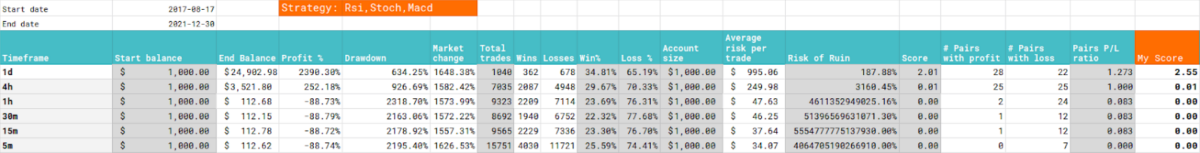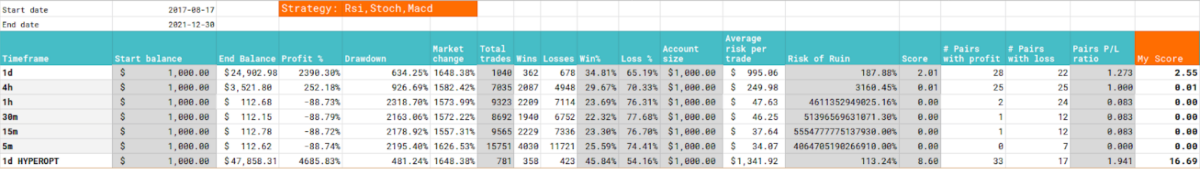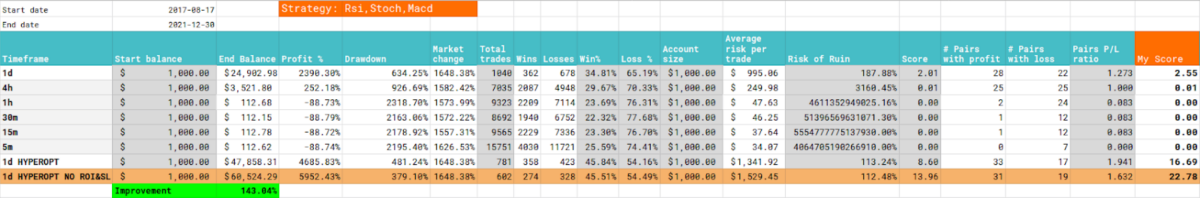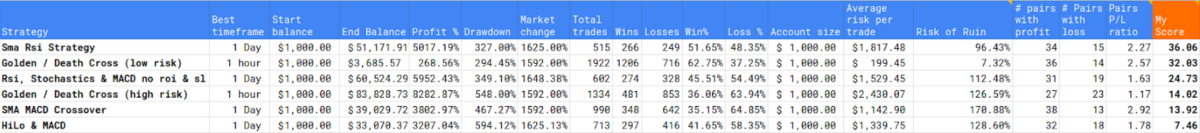# Rsi, Stochastics & MACD aka. PowerX strategy - Is this strategy profitable or not?

### Intro

In this blog series I will be searching for the best trading strategy for cryptocurrency trading with a trading bot. These posts are complementary to the video's I make on Youtube, which you can watch below.

The trading bot I use is the Freqtrade (https://www.freqtrade.io/en/latest/) trading bot which not only has good options for bot trading, but also is excellent in backtesting and optimization of trading parameters. Therefore I will use this program to not only trade automatically, but also look for the best strategies and setups.

All code that is specifically written for this strategy or for hyperparameter optimisation is available at the bottom of this post.

If you appreciate the effort I take to create this strategy or optimisation code, then please consider a tip for this post!

### The backtesting setup

If you want to know more on how I do these backtests like: which pairs I use, which timeframes and which periods I test, then see this blog post to know more about the approach I use: https://www.publish0x.com/dutchcryptodad/is-it-profitable-or-not-the-setup-and-approach-xppgjoj

### Strategy

This time I am going to test a combination of the RSI, Stochastics and MACD.

This strategy is simple and can be coded to do automatic trading with it.

The strategy is as follows:

• When the RSI is above the 50 level
• The Stochastics %D is above the 50 level
• And the MACD histogram is above the zero line
• Then a buy signal is generated.

From this point on I will diverge a littlebit from the original proposed strategy with these indicators because the stoploss and exit signals are based on ATR and the entry point

This is not a default option of the Bot I am using so therefore I will keep things a little bit more simple. I will base my exit signals on the default capabilities of the trading bot

So RSI should be below 50, %D should be below 50 and MACD histogram should be below 0.

### Stoploss and takeprofit

I will run a first backtest where the exit signals are based on the initial entry indicators. Here I will see what the results are on the multiple timeframes.

Then I will let Hyperopt determine the stoploss and takeprofit points based on percentages and time.

### Initial backtest results

After backtesting, the initial backtest results are as follows:

• The best timeframe is the 1 day timeframe.
• It has a backtestes profit of 2390 percent which could have turned 1000 dollar into under 25000 dollar hypothetically
• The drawdown is 634 percent and the possible risk of ruin is 187 percent.
• Again, this is based on a slight adjustment of the original strategy where the exits are based on the atr and a risk reward of 1 to 2 is advised.### Hyperparameter optimisation

In the Hyperopt session I wil ltry to find the optimal settings for the following parameters:

• The RSI horizontal Buy line threshold
• The SlowD horizontal Buy line threshold

After this session, there will be another backtest with the optimised parameters on the given timeframe and range to see if this strategy has improved or not.As you can see the hyperopt session with proposed ROI, Stoploss and adjusted boundaries for RSI and %D gave some some good improvements.

It improved the original strategy with more than 90%

### Conclusions

I am always curious to find out if I can tweak some things even further. So I did a second hyperopt with the intention to let this strategy ride the trend instead of taking profit at a certain point in time.

Also I wanted to find out which indicators proved to give the best exit signals. So what I did is backtest a couple of times with only one exit indicator.

This way I found out that when the stochastic %D indicator got below its entry boundary, it gave the exit signal with the highest profit.

By doing this I could even improve the results of this strategy even further and got to a profit of 5952 percent with less drawdow.

And the nice thing is that My winrate and risk of ruin were almost similar to this strategy with the use of ROI and stoploss activated.

But be aware that by doing this I have curve fitted this strategy a little bit too much. So take this in mind when you do your own research on this strategy!• Best timeframe: 1 day
• No Stoploss and ROI
• Use RSI > 65 AND Stoch %D > 69
• Exit indicator is the stochastics %D < 69
• If you started with 1000 USDT, you now could have 60500 USDT
• Total profit of strategy: 5952 %
• Winrate: 45 %
• Drawdown of strategy: 349  %
• Risk of ruin of strategy: 112 %

Nonetheless I have found that this strategy is a good performer when you just let it ride the trends.

It gives you nice buy signals based on three different indicators. You can always follow the original settings and rules of the strategy. That means that you use takeprofit and use stoploss based on the ATR. But with backtest and parameter optimisation it got better results if you adjust the rsi boundary from 50 to 65 and the %D boundary of the stochastics from 50 to 69. Also the exit signal based on the %D proved to give the best returns.

### Strategy League

If I enter the results of this test in the overall sheet then i come to the conslusion that this strategy takes the third place overall. It has a higher profit percentage than the top two but became third because of the lower winrate and drawdown. But considering the other strategies this looks like a nice strategy to follow.### The strategy code

``````# --- Do not remove these libs ---
from pandas import DataFrame

import talib.abstract as ta

class powerx(IStrategy):
# Trading strategy based on Markus Heitkoetter's PowerX strategy
stoploss = -100.0
timeframe = "1d"
# trailing_stop = True
# trailing_stop_positive = 0.3
minimal_roi = {"0": 100.}

order_types = {
"sell": "limit",
"emergencysell": "market",
"stoploss": "market",
"stoploss_on_exchange": True,
"stoploss_on_exchange_interval": 60,
"stoploss_on_exchange_limit_ratio": 0.99,
}

plot_config = {
# Main plot indicators (Moving averages, ...)
"main_plot": {
"sma": {},
},
"subplots": {
# Subplots - each dict defines one additional plot
"MACD": {
"macd": {"color": "blue"},
"macdsignal": {"color": "orange"},
},
"RSI": {
"rsi": {"color": "red"},
},
"STOCH": {
"slowd": {"color": "red"},
},
},
}

def populate_indicators(self, dataframe: DataFrame, metadata: dict) -> DataFrame:
# SMA
dataframe["SMA"] = ta.SMA(dataframe, timeperiod=20)
# RSI
dataframe["rsi"] = ta.RSI(dataframe, timeperiod=7)
# SLOW STOCHASTIC
# https://mrjbq7.github.io/ta-lib/doc_index.html
stoch = ta.STOCH(
dataframe,
fastk_period=14,
slowk_period=3,
slowk_matype=0,
slowd_period=3,
slowd_matype=0,
)
dataframe["slowd"] = stoch["slowd"]
dataframe["slowk"] = stoch["slowk"]
# MACD
# https://mrjbq7.github.io/ta-lib/func_groups/momentum_indicators.html
macd = ta.MACD(
dataframe,
fastperiod=12,
fastmatype=0,
slowperiod=26,
slowmatype=0,
signalperiod=9,
signalmatype=0,
)
dataframe["macd"] = macd["macd"]
dataframe["macdsignal"] = macd["macdsignal"]
dataframe["macdhist"] = macd["macdhist"]

# print(dataframe.tail(20))
return dataframe

# pass
dataframe.loc[
(
(dataframe["rsi"] > 50)
& (dataframe["slowd"] > 50)
& (dataframe["macdhist"] > 0)
),
] = 1
return dataframe

def populate_sell_trend(self, dataframe: DataFrame, metadata: dict) -> DataFrame:
# pass
dataframe.loc[
(
(dataframe["rsi"] < 50)
| (dataframe["slowd"] < 50)
| (dataframe["macdhist"] < 0)
),
"sell",
] = 1
# print(dataframe.tail(20))
return dataframe``````

### The hyperopt code

``````# Commands
# freqtrade hyperopt -c user_data/backtest-config.json -s powerxhopt --epochs 50 --spaces buy roi stoploss --hyperopt-loss SharpeHyperOptLossDaily
# freqtrade backtesting -c user_data/backtest-config.json -s powerxhopt

# --- Do not remove these libs ---
import talib.abstract as ta
import pandas_ta as pta
import numpy as np  # noqa
import pandas as pd  # noqa
from pandas import DataFrame
from functools import reduce
from freqtrade.strategy import (BooleanParameter, CategoricalParameter, DecimalParameter,IStrategy, IntParameter)

class powerxhopt(IStrategy):
# Trading strategy based on Markus Heitkoetter's PowerX strategy
stoploss = -100.0
timeframe = "1d"
minimal_roi = {"0": 100.}

order_types = {
"sell": "limit",
"emergencysell": "market",
"stoploss": "market",
"stoploss_on_exchange": True,
"stoploss_on_exchange_interval": 60,
"stoploss_on_exchange_limit_ratio": 0.99,
}

plot_config = {
# Main plot indicators (Moving averages, ...)
"main_plot": {
"sma": {},
},
"subplots": {
# Subplots - each dict defines one additional plot
"MACD": {
"macd": {"color": "blue"},
"macdsignal": {"color": "orange"},
},
"RSI": {
"rsi": {"color": "red"},
},
"STOCH": {
"slowd": {"color": "red"},
},
},
}

# --- Define spaces for the indicators ---
rsi_hline = IntParameter(30, 70, default=50, space="buy")
stoch_hline = IntParameter(30, 70, default=50, space="buy")

def populate_indicators(self, dataframe: DataFrame, metadata: dict) -> DataFrame:
# SMA
dataframe["SMA"] = ta.SMA(dataframe, timeperiod=20)
# RSI
dataframe["rsi"] = ta.RSI(dataframe, timeperiod=7)
# SLOW STOCHASTIC
# https://mrjbq7.github.io/ta-lib/doc_index.html
stoch = ta.STOCH(
dataframe,
fastk_period=14,
slowk_period=3,
slowk_matype=0,
slowd_period=3,
slowd_matype=0,
)
dataframe["slowd"] = stoch["slowd"]
dataframe["slowk"] = stoch["slowk"]
# MACD
# https://mrjbq7.github.io/ta-lib/func_groups/momentum_indicators.html
macd = ta.MACD(
dataframe,
fastperiod=12,
fastmatype=0,
slowperiod=26,
slowmatype=0,
signalperiod=9,
signalmatype=0,
)
dataframe["macd"] = macd["macd"]
dataframe["macdsignal"] = macd["macdsignal"]
dataframe["macdhist"] = macd["macdhist"]
# print(dataframe.tail(20))
return dataframe

# pass
conditions = []
conditions.append(
(dataframe['rsi'] > self.rsi_hline.value )
& (dataframe['slowd'] > self.stoch_hline.value )
& (dataframe["macdhist"] > 0)
)

if conditions:
dataframe.loc[
reduce(lambda x, y: x & y, conditions),

return dataframe

def populate_sell_trend(self, dataframe: DataFrame, metadata: dict) -> DataFrame:
# The PowerX strategy works on R:R 2:1 take profit therefore exits based on indicators
# is actually not the official way to take profit
# pass
conditions = []
conditions.append(
# (dataframe['rsi'] < self.rsi_hline.value )
# Playing with these exit indicators proved that using only the slowd
# crossing had a higher profit than using the three indicators together
# or separate but only when RSI > 34 and slowd > 68
(dataframe['slowd'] < self.stoch_hline.value )
# | (dataframe["macdhist"] < 0)
)

if conditions:
dataframe.loc[
reduce(lambda x, y: x & y, conditions),
'sell'] = 1

return dataframe``````

### The hyperopt json results

``````{
"strategy_name": "powerxhopt",
"params": {
"roi": {
"0": 100.0
},
"stoploss": {
"stoploss": -100.0
},
"trailing": {
"trailing_stop": false,
"trailing_stop_positive": null,
"trailing_stop_positive_offset": 0.0,
"trailing_only_offset_is_reached": false
},
"rsi_hline": 65,
"stoch_hline": 69
},
"sell": {},
"protection": {}
},
"ft_stratparam_v": 1,
"export_time": "2022-01-17 11:05:10.331653+00:00"
}``````I'm just a regular Dutch dad with a passion for crypto, trading, technology and learning. This channel is my personal journey into the world of Crypto, blockchain, programming, trading bots, trading strategies, NFT's, Defi and many things more.